# KSEEB Solutions for Class 7 Maths Chapter 11 Perimeter and Area Ex 11.4

Students can Download Chapter 11 Perimeter and Area Ex 11.4, Question and Answers, Notes Pdf, KSEEB Solutions for Class 7 Maths, Karnataka State Board Solutions help you to revise complete Syllabus and score more marks in your examinations.

## Karnataka State Syllabus Class 7 Maths Chapter 11 Perimeter and Area Ex 11.4

Question 1.
A garden is 90 m long and 75 m broad. A path 5 m wide is to be built outside and around it. Found the area of the path. Also find the area of the garden in hectare.
Solution: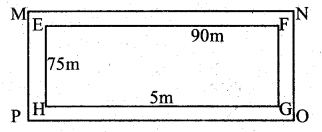Area of the garden = l × b
Length = l = 90 m
Breath = b = 75 m
∴ Area of the garden EFG ||)} = 90 × 75
= 6750 sq mtrs
1 Hectare = 10,000 sqmts.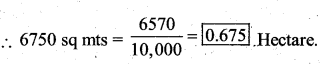Area of the outer skirt of the garden = l × b
length = 90 + 5 + = 100 m
breadth = 75 + 5 + 5 = 85 m
∴ Area = 100 × 85 = 8,500sqm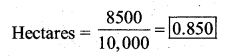Area of the path = 0.850 – 0.675 = 0.175
0.175 hectare or 1750 sq mts

Question 2.
A 3 m wide path runs outside and around a rectangular park of length 125 m and breadth 65 m. Find the area of the path.
Solution:
length = l = 125 breadth = b = 65 m
width of a path = 3 m
Area of a rectangular park = l × b = 125 × 65
= 8125 sq mts
Area of ABCD = l × b
l = 125 + 3 + 3 = 131 b = 65 + 3 + 3 = 71
∴ Area = 131 × 71 = 9301 sq mts
Area of the path = Area of ABCD – Area
of park 9301 – 8125 = 1176 sq mts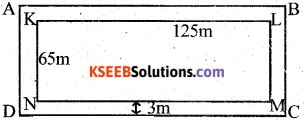Question 3.
A picture is painted on a cardboard 8 cm long and 5 cm wide such that there is a margin of 1.5 cm along each of its sides. Find the total area of the margin.
Solution: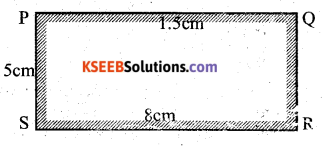Area of cardboard = l × b
l = 8 cm b = 5 cm Margin = 1.5 cm
Area of picture = l × b
l = 8 = (1.5 + 1.5) = 5 cms b = 5 = (1.5 + 1.5) = 2 cms
Area = l × b = 5 × 2
Area of margin = Area of Cardboard – Area of picture
= 40 – 10 = 30 sq cmsQuestion 4.
A verandah of width 2.25 m is constructed all along outside a room which is 5.5 m long and 4 m wide. Find the area of the verandah: the cost of cementing the floor of the verandah at the rate of ₹ 200 per m2. The area of the verandah with the room. (Fig KLMN)
Solution:
l = length = 5.5 + 2.25 + 2.25 = 10 m
b = breadth = 4 + 2.25 + 2.25 = 8.5 m
∴ Area 10 × 8.5
= 85 sq cms.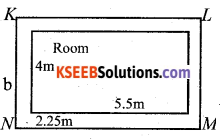Area of room = l × b
l = 5.5 m = 5.5 × 4 N
∴ Area of verandah = Area of KLMN – Area of room
= 85 – 22 = 63 sq mts
Cost of cementing the floor = Rs 200 / sq m
Total cost of cementing = 63 × 200 = Rs 12,600

Question 5.
A path 1 m wide is built along the border and inside a square garden of side 30m. Find :
i) the area of the path
ii) the cost of planting grass in the remaining portion of the garden at the rate of ₹ 40 per m2.
Solution: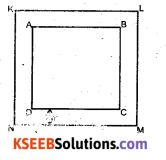Area of KLMN = side × side = 30 × 30
Area of ABCD = 30 – (1 + 1) = 28
= 28 × 28
= 784 sq mts
Area of the path = Area of KLMN – Area of ABCD
Cost of planting grass = Rs 40 / sq m
∴ Total cost of planting = 784 × 40
= Rs 31.360Question 6.
Two cross roads, each of width 10 m, cut at right angles through the centre of a rectangular park of length 700 m and breadth 300 m and parallel to its sides. Find the area of the roads. Also find the area of the park excluding cross roads. Give the answer in hectares.
Solution: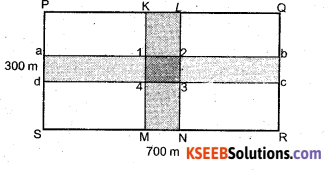Area of PQRS = l x b = 700 × 300
= 2,10,000 sq m
Area of cross Roads = Area of KLMN abcd – 1, 2, 3, 4
= 300 × 10 + 700 × 10 – 10 × 10
= 3000 + 7000 – 100
= 10,000 – 100 = 9,900 sq mts
Area of the park excluding the cross roads = 2,10,000 – 9,900
= 2,00,100 sq mts.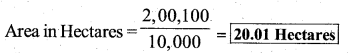Question 7.
Through a rectangular filed of length 90 m and breadth 60 m, two roads are constructed which are parallel to the sides and cut each other at right angles through the centre of the fields. If the width of each road is 3 in. Find :
i) The area covered by the roads.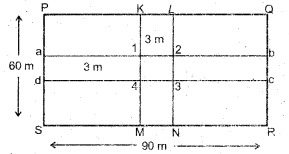Area covered by the roads = Area of klmn + Area of abcd – Area of 1, 2, 3, 4
Area of abcd = 90 × 3 = 270 sq mtrs
Area of klmn = 60 × 3 = 180 sq mts.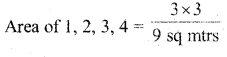= 180 + 270 – 9 = 441 sq mts.

ii) the cost of constructing the roads at the rate of ₹ 110 per m2.
Cost of constructing the roads = Rs 110/sq m
∴ Total cost of constructing the roads
= 441 × 110 = Rs. 48.510Question 8.
Pragya wrapped a cord around a circular pipe of radius 4 cm (adjoining figure)
and cut off the length required of the cord. Then she wrapped it around a square box of side 4 cm (also shown).
Did she have any cord left? (r = 3.14)
Solution: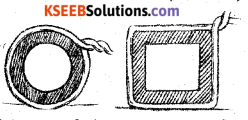Radius of the circular pipe} r = 4 cm
Cirumference of the circular pipe } 2πr
=2 × 3.14 × 4
= 25.12 cms
Perimeter of the square box = 4 × side
= 4 × 4
= 16 cms
yes, the length of the cord left = 25.12 – 16
= 9.12 cms

Question 9.
The adjoining figure represents a rectangular lawn with a circular flower bed in the middle. Find
Solution: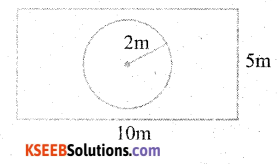i) the area of the whole land
Area of the whole land = l × b
l = 10 mts = 10 × 5
b = 5 mts = 50 sq mts

ii) the area of the flower bed
Area of the flower bed = πr2
r = 2m = 3.14 × 2 × 2
= 12.56 sq mtsiii) the area of the lawn excluding the area of the flower bed
Solution:
The area of the lawn excluding the circular flower bed } = 50 – 12.56
= 37.44 sq m

iv) the circumference of the flower bed.
The circumference of the circular flower bed} = 2πr
= 2 × 3.14 × 2
= 12.56 mts.

Question 10.
In the following figures, find the area of the shaded portions :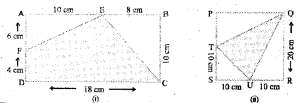Solution:
1) Area of the rectangle ABCD = l × b
l = 18 cms = 18 × 10
b = 10 cms = 180 sq cms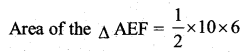= 30 sq cms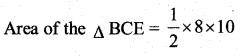= 40 sq cms
Area of the shaded position = Area of rectangle ABCD – (AreaofAEF+AreaofBCE)
= 180 – (30 + 40)
= 180 – 70 = 1110 sq cms

ii) Area of the square PQRS = side × side
= 20 × 20
= 400 sq cms.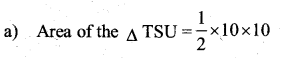= 50 sq cm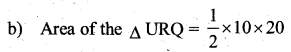= 100 sq cms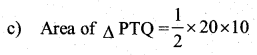= 100 sq cm
∴ Area of shaded region = 400 – (50 + 100 – 100)
= 400 – 250
= 150 sq cms.Question 11.
Find the area of the quadrilateral ABCD.
Here, AC = 22 cm, BM = 3 cm, DN = 3 cm, and BM ⊥ AC, DN ⊥ AC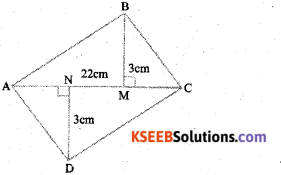Solution:
Area of the quadrilateral ABCD =
Area of the ∆ ABC + Area of the ∆ ACD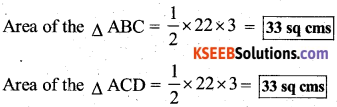∴ Area of quadrilateral ABCD = 33 + 33
= 66 sq cms